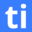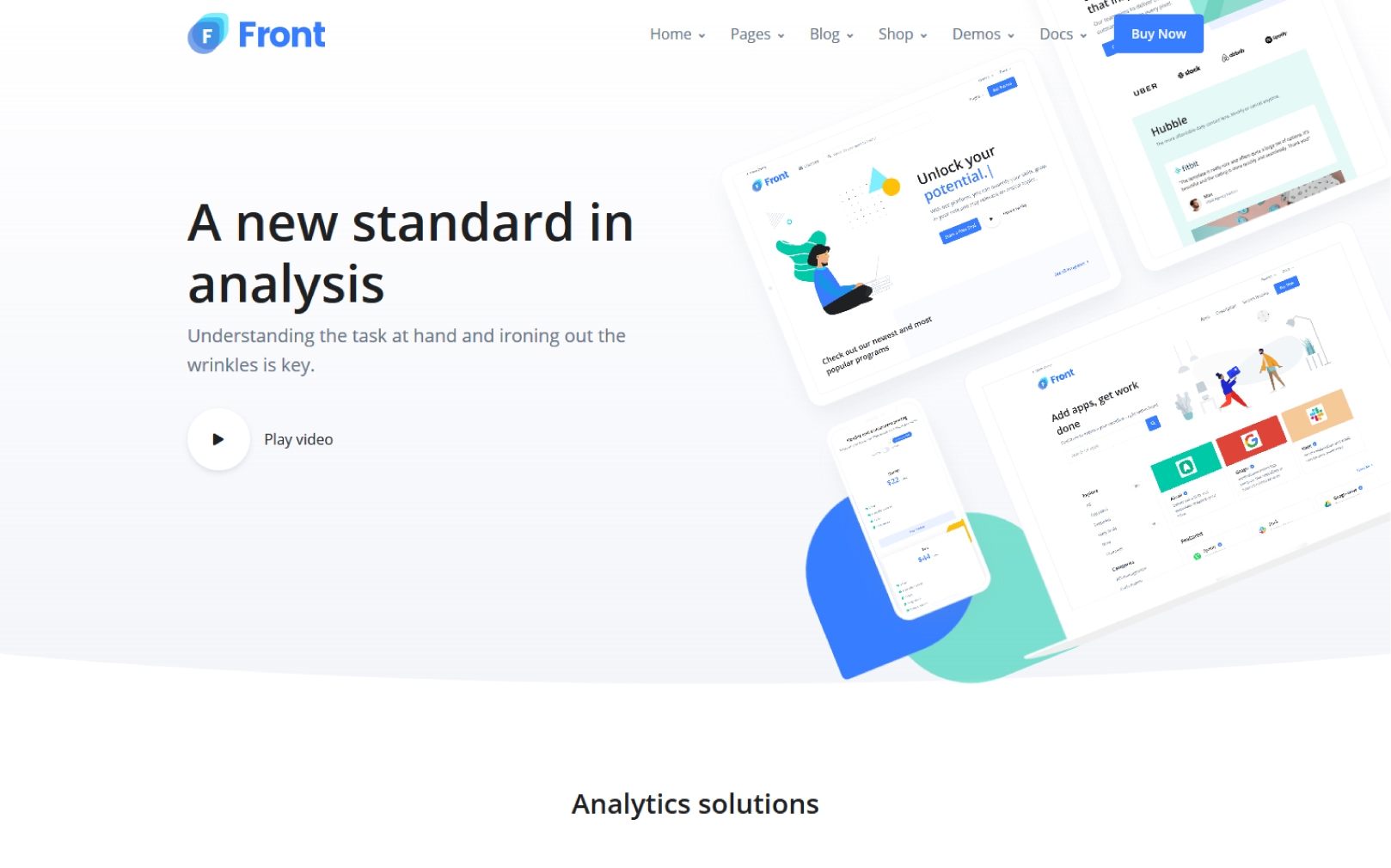# Utilize the Best Online Numerical Problem Solving Test for Recruitment

Testinvite Talent Numerical Problem Solving Test evaluates the ability to find a solution to a problem by applying numerical techniques. The test consists of various types of word problems that involve mixed math operations. Numerical reasoning abilities and basic mathematical knowledge are both needed to answer questions. Testinvite Talent Numerical Problem-Solving Test scores can assist employers to understand candidates' ability to form strategies and implement solutions to problems.Created by Testinvite

10 mins

7

Intermediate

English

## About the Numerical Problem Solving Test (Intermediate)

Mostly used for

Mathematical knowledge is characterized by the logical relationship based on mathematical information. Creating these logical relationships is a part of understanding and learning mathematical knowledge. Understanding mathematical knowledge and establishing the relationship between this knowledge can occur in the numerical problem-solving process. Numerical problem solving is an integrative mathematical process that involves finding a solution to a problem by applying numerical techniques. It can be used to find the best solution for a given problem or it can be used as an exploratory tool for understanding the behavior of mathematical models.

Testinvite Talent Numerical Problem-Solving Test is based on various types of word problems that involve mixed math operations. A word problem is a way of presenting mathematical exercises in ordinary language rather than in mathematical notation. Therefore, word problems require candidates to apply their knowledge of various concepts to 'real-life' scenarios. To succeed in Testinvite Talent Numerical Problem-Solving Test, it is needed to develop efficient reasoning abilities that allow solving problems without spending too much time. It is also needed to be familiar with essential parts of basic mathematics and mental arithmetic.

Numerical problem-solving abilities are often needed in fields of engineering, science, medicine, finance where it is necessary to find the best solution for a given problem or understand the behavior of mathematical models. Testinvite Talent Numerical Problem-Solving Test is suitable for Accountants, Architects, Biologists. Medical Doctors, Chemists. Nurses. Tradesmen, Lawyers, Psychologists recruitment process.

In mathematics, the reasoning is more crucial than performing mathematical operations based on memorization and repetition. This need has also manifested itself in problem-solving, which is one of the most difficult subjects to perform in mathematics. In the rapidly changing work environment, problem-solving has been interspersed in almost every subject. Consequently, candidates are expected to become more active in problem-solving processes.

### Start analytical screening for hiring!

Why Testinvite talent solutions?

## Make great hiring decisions, fast.## FAQs

We recommend combining Testinvite’s Talent Core Skill Tests to create the best assessment solution for your recruitment process. You can add up to six tests to each assessment.

There are no extra charges or hidden fees in Test Invite. Testinvite has a transparent credit-based system that allows you to pay only for what you use. You can find pricing details here.

Testinvite safe assessment browser enables you to conduct secure assessments and protect the full integrity of online exams. You can find detailed information about how to enhance security and which security measures you need to take here.

Yes, validity and reliability are at the core of our test development methodology. We use advanced statistical methods to make sure the test has validity and reliability. You can find detailed information about our methodology here.

To solve a numerical test, it is needed to be familiar with essential parts of basic mathematics and mental arithmetic.